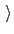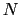Next: Multidimensional histograms Up: Biasing and analysis methods Previous: Linear restraints   Contents   Index

## Adaptive Linear Bias/Experiment Directed Simulation

Experiment directed simulation applies a linear bias with a changing force constant. Please cite White and Voth  when using this feature. As opposed to that reference, the force constant here is scaled by the width corresponding to the biased colvar. In White and Voth, each force constant is scaled by the colvars set center. The bias converges to a linear bias, after which it will be the minimal possible bias. You may also stop the simulation, take the median of the force constants (ForceConst) found in the colvars trajectory file, and then apply a linear bias with that constant. All the notes about units described in sections 13.5.5 and 13.5.4 apply here as well. This is not a valid simulation of any particular statistical ensemble and is only an optimization algorithm until the bias has converged.

• name: see definition of name (biasing and analysis methods)
• colvars: see definition of colvars (biasing and analysis methods)

• centersCollective variable centersContext: alb
Acceptable values: space-separated list of colvar values
Description: The desired center (equilibrium values) which will be sought during the adaptive linear biasing. The number of values must be the number of requested colvars. Each value is a decimal number if the corresponding colvar returns a scalar, a (x, y, z)'' triplet if it returns a unit vector or a vector, and a q0, q1, q2, q3)'' quadruplet if it returns a rotational quaternion. If a colvar has periodicities or symmetries, its closest image to the restraint center is considered when calculating the linear potential.
• updateFrequencyThe duration of updatesContext: alb
Acceptable values: An integer
Description: This is,, the number of simulation steps to use for each update to the bias. This determines how long the system requires to equilibrate after a change in force constant (), how long statistics are collected for an iteration (), and how quickly energy is added to the system (at most,, whereis the forceRange). Until the force constant has converged, the method as described is an optimization procedure and not an integration of a particular statistical ensemble. It is important that each step should be uncorrelated from the last so that iterations are independent. Therefore,should be at least twice the autocorrelation time of the collective variable. The system should also be able to dissipate energy as fast as, which can be done by adjusting thermostat parameters. Practically,has been tested successfully at significantly shorter than the autocorrelation time of the collective variables being biased and still converge correctly.

• forceRangeThe expected range of the force constant in units of energyContext: alb
Acceptable values: A space-separated list of decimal numbers
Default value: 3Description: This is largest magnitude of the force constant which one expects. If this parameter is too low, the simulation will not converge. If it is too high the simulation will waste time exploring values that are too large. A value of 3has worked well in the systems presented as a first choice. This parameter is dynamically adjusted over the course of a simulation. The benefit is that a bad guess for the forceRange can be corrected. However, this can lead to large amounts of energy being added over time to the system. To prevent this dynamic update, add hardForceRange yes as a parameter
• rateMaxThe maximum rate of change of force constantContext: alb
Acceptable values: A list of space-separated real numbers
Description: This optional parameter controls how much energy is added to the system from this bias. Tuning this separately from the updateFrequency and forceRange can allow for large bias changes but with a low rateMax prevents large energy changes that can lead to instability in the simulation.Next: Multidimensional histograms Up: Biasing and analysis methods Previous: Linear restraints   Contents   Index
vmd@ks.uiuc.edu